Year 8 Interactive Maths - Second Edition

## Basic Operations

###### Recall:

The four basic mathematical operations are:Adding two (or more) numbers means to find their sum (or total).  The symbol used for addition is '+'.

For example, 5 + 10 = 15

This is read as five plus ten is equal to fifteen or simply, five plus ten is fifteen.

#### Example 1

Find the sum of 9 and 8.

##### Solution:

9 + 8 = 17

To add large numbers, list them in columns and then add only those digits that have the same place value.

#### Example 2

Find the sum of 5897, 78, 726 and 8569.

##### Solution:###### Note:
• Write the numbers in columns with the thousands, hundreds, tens and units lined up.
• 7 + 8 + 6 + 9 = 30.  Thus, the sum of the digits in the units column is 30.  So, we place 0 in the units place and carry 3 to the tens place.
• The sum of the digits in the tens column after adding 3 is 27.  So, we place 7 in the tens place and carry 2 to the hundreds place.
• The sum of the digits in the hundreds column after adding 2 is 22.  So, we place 2 in the hundreds place and carry 2 to the thousdands place.

### Subtraction

Subtracting one number from another number is to find the difference between them.  The symbol used for subtraction is '–'.  This is known as the minus sign.

For example, 17 – 8 = 9

This is read as seventeen take away eight is equal to nine (or seventeen take away eight is nine).  Also, we can say that 17 minus 8 is 9.

#### Example 3

Subtract 9 from 16.

16 – 9 = 7

### Subtraction of Large Numbers

To subtract large numbers, list them in columns and then subtract only those digits that have the same place value.

#### Example 4

Find the difference between 7064 and 489.

##### Solution: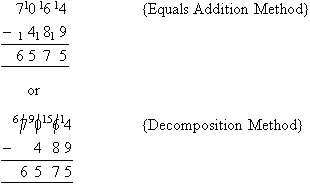###### Note:
• Use the equals addition method or the decomposition method.
• Line up the thousands, hundreds, tens and units place values for the two numbers when placing the smaller number below the larger number as shown above.

### Multiplication

Multiplication means times (or repeated addition).  The symbol used for multiplication is '×'.

For example, 7 × 2 = 14
This is read as seven times two is equal to fourteen or simply, seven times two is fourteen.

To multiply a large number with another number, we write the numbers vertically and generally multiply the larger number with the smaller number.

###### Note:

A product is the result of the multiplication of two (or more) numbers.

#### Example 5

Calculate 765 × 9.

##### Solution:

Write the smaller number, 9, under the larger number, 765, and then calculate the multiplication.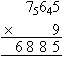###### Note:
• 9 × 5 = 45.  So, place 5 units in the units column and carry the 4 (i.e. four tens) to the tens column.
• Calculate 9 × 6 and then add 4 to give 58 (i.e. 58 tens).  Then place 8 in the tens column and carry 5 to the hundreds column.
• Finally multiply 7 by 9 and add 5 to give 68 (i.e. 68 hundreds).  Write this number down as shown above.
###### Remember:
• To multiply two large numbers, write the numbers vertically with the larger number generally being multiplied by the smaller number which is called the multiplier.
• We use the 'times table' to find the product of the larger number with each digit in the multiplier, adding the results.
• Remember to add a zero for every place value after the multiplying digit.   For example, if the multiplying digit is in the hundreds column, add two zeros for the tens column and for the units column.

#### Example 6

Calculate 38 × 70.

##### Solution: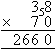###### Note:
• Multiplying 38 by 70 is quicker than multiplying 70 by 38 as 70 contains a zero.
• A zero is placed in the units column.  Then we calculate 7 × 38 as shown above.

#### Example 7

Calculate 385 × 500.

##### Solution:###### Note:
• Multiplying 385 by 500 is quicker than multiplying 500 by 385 as 500 contains two zeros.
• A zero is placed in the units column and also the tens column.  Then we calculate 5 × 385 as shown above.

#### Example 8

Calculate 169 × 68.

##### Solution:###### Note:
• To multiply 169 by 68, place 68 below 169.
• Then we calculate 8 × 169 and 60 × 169 as shown above.

### Division

Division 'undoes' multiplication and involves a number called the dividend being 'divided' by another number called the divisor.  The symbol used for division is '÷'.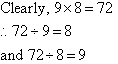#### Example 9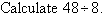##### Solution: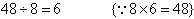#### Example 10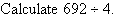##### Solution: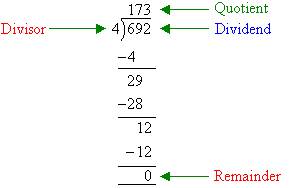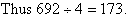###### Note:
• As division is the inverse of multiplication, start by dividing 4 into the column furthest to the left.
• 6 ÷ 4 = 1 and 2 is the remainder.
• Clearly, the remainder 2 is 200 (i.e. 20 tens); and we can carry this into the tens column to make 29.
• Now, 29 ÷ 4 = 7 with a remainder of 1.  Clearly, the remainder of 1 is 10 (i.e. 10 units) and we carry this into the units column to make 12.
• Finally, 12 ÷ 4 = 3.

#### Example 11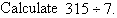##### Solution: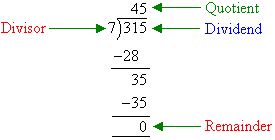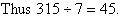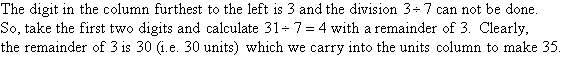###### Summary
• The four basic mathematical operations are:• Adding two (or more) numbers means to find their sum (or total).
• Subtracting one number from another number is to find the difference between them.
• Multiplication means times (or repeated addition).  A product is the result of the multiplication of two (or more) numbers.
• Division 'undoes' multiplication.